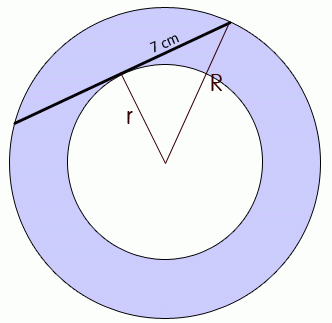SEARCH HOMEMath Central Quandaries & QueriesQuestion from James, a student: Hi, Can you please show me to work out this problem? Problem: Two concentric circles have a chord running through the outer one. The chord is the tangent of the inner circle and is 14 cm.The outer circle is shaded and the inner circle is not. Find the exact area of the shaded region without using a calculator.Hi James.

Here is a diagram I drew of your problem. I've also drawn the radius of the outer circle as R and the radius of the inner circle as r. Remember that at the point of tangency, the tangent line is perpendicular to the radius of a circle, so this triangle is a right-angled triangle. As well, symmetry requires that the side of the right triangle be half of the chord length, so I've labelled it 7 cm.The area of the outer circle is πR2 .
The area of the inner circle is πr2 .
Therefore the area of the shaded region is the difference of the two:

A = π(R2 - r2)

Since that is a right-triangle, Pythagorus applies, so

R2 = 72 + r2
R2 - r2 = 72

Thus,

A = π(72)

Hope this helps,
Stephen La Rocque.Math Central is supported by the University of Regina and The Pacific Institute for the Mathematical Sciences.